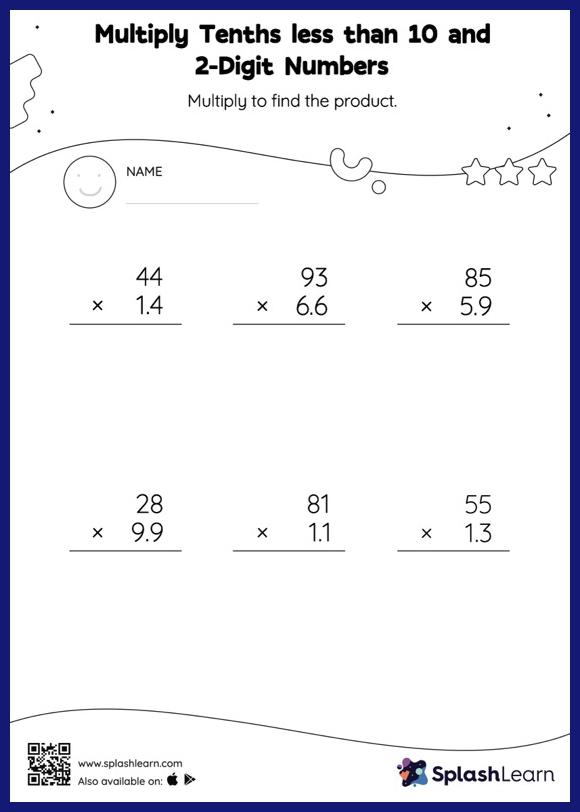# Multiply Tenths less than 10 and 2-Digit Numbers: Vertical Multiplication Worksheet

Home > Multiply Tenths less than 10 and 2-Digit Numbers: Vertical MultiplicationTask your little mathematicians to crack the code of multiplying tenths less than 10 and 2-digit numbers with this fun worksheet. Students may omit the decimal point when multiplying a decimal by a two-digit number and then add it when writing the answer. This multiply tenths less than 10 and 2-digit numbers worksheet gives learners many opportunities to practice this concept. In this worksheet, students practice solving problems using the column method. This method is especially helpful with problems involving bigger multi-digit numbers as the format provides an easy structure to follow.